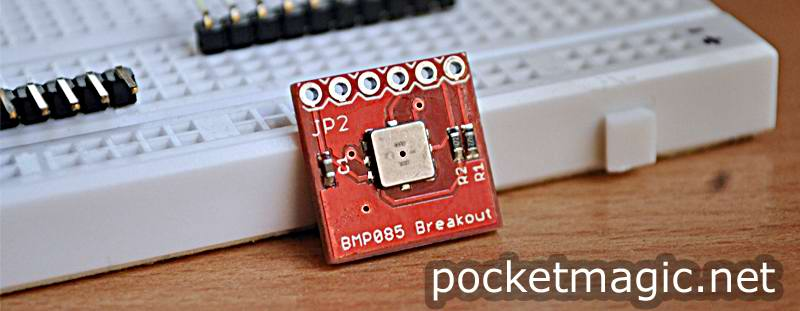# BMP085 Barometric pressure sensorI needed to add pressure reading capabilities to my radiation monitoring station, uRadMonitor. For barometric pressure, there aren’t many options when it comes to sensors. I stumbled upon the Bosch BMP085 sensor, described as a highly sensitive sensor that can sense differences for each 9cm of an air column. Besides pressure, it also offers temperature data, all packed nicely in a compact module.
The only “problem” is that it provides digital data output, via the i2c bus. My other sensors where not using it, so I had to add the i2c code solely for this sensor, resulting in higher software size.

Key features:
Pressure range: 300..1100hPa (+9000m … -500m above sea level)
Supply voltage: 1.8..3.6V (Vdda) 1.62V..3.6V (Vddd)
Low power: 5uA at 1sample/sec in standard mode
Low noise: 0.06hPa (0.5m) in ultra low power mode ; 0.03hPa (0.25m) ultra high resolution mode
Operating temperature: operational in -40..+85°C, with full accuracy in 0..+65°C
Resolution of output data: 1Pa for pressure, 0.1°C for temperature
Complete datasheet here.

On Earth, standard atmospheric pressure is 101 325 Pa. Meteorological barometric pressure reports typically report atmospheric pressure in hectopascals. More here. The BMP085 returns the measured pressure in Pascals and the temperature in degrees Celsius.

The BMP085 is designed to be connected directly to a microcontroller via the i2c bus. The pressure and temperature data has to be compensated by the calibration data of the EPROM of the BMP085. Reading the calibration done must be done only once, before reading the pressure or temperature data:

```void getcalibration() {
uint8_t buff;
memset(buff, 0, sizeof(buff));
ac1 = ((int)buff <<8 | ((int)buff));
ac2 = ((int)buff <<8 | ((int)buff));
ac3 = ((int)buff <<8 | ((int)buff));
ac4 = ((unsigned int)buff <<8 | ((unsigned int)buff));
ac5 = ((unsigned int)buff <<8 | ((unsigned int)buff));
ac6 = ((unsigned int)buff <<8 | ((unsigned int)buff));
b1 = ((int)buff <<8 | ((int)buff));
b2 = ((int)buff <<8 | ((int)buff));
mb = ((int)buff <<8 | ((int)buff));
mc = ((int)buff <<8 | ((int)buff));
md = ((int)buff <<8 | ((int)buff));
}
```

I used a BMP085 breakout board, that already has thee 4.7k pull-up resistor on the SDA and SCL pins.

Knowing the pressure it is possible to compute the altitude. A convenient alternative to GPS!

```// we assume sea level pressure is 101325 Pa
float convertAltitude(int32_t pressure) {
return ((1 - pow(pressure/(double)101325, 0.1903 )) / 0.0000225577);
}
```

Here is the code, based on Davide Gironi's work on the BMP085, and the i2c interface originally developed by Peter Fleury.
BMP085